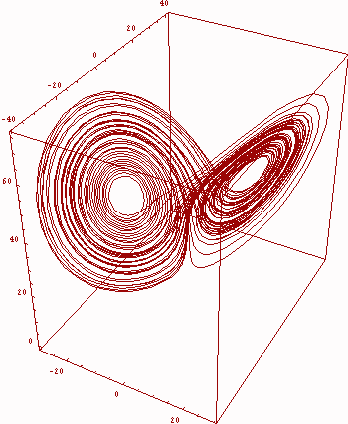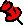Page 4 Additional Applications... A few nice Integrals fun with Dirac Gamma Matrices Dirac Equation manipulations Random Walks Spherical Harmonics                                                                Notebook       Spherical Bessel Functions                                                      Notebook       Elliptic Functions                                                                      Notebook       Hypergeometric Functions                                                        Notebook       Pade Approximants                                                                 Notebook       Solution of the Schroedinger Eq. (Harm. Oscillator)         Notebook       Solution of the radial Schroedinger Eq. (Yukawa pot.)     Notebook       Schwarzschild metric                                                                 Notebook       Robertson-Walker metric                                                         Notebook